## Symbolic Algebra

Cassiopeia uses MathML content markup to represent equations internally. Equations thus have mathematical meaning and can therefore be processed by the built-in symbolic algebra system.

### Taking derivatives

Press Command-y to create a new equation and then type "y", "=", "a", Ctrl-l, "3", <Cursor-up>, <Cursor-right>, "x", Ctrl-h, "3", <Cursor-up>, <Cursor-right>, "+", "a", Ctrl-l, "2", <Cursor-up>, <Cursor-right>, "x", Ctrl-h, "2", ...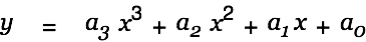Now Command-Shift-Doubleclick on this equation to open the equation inspector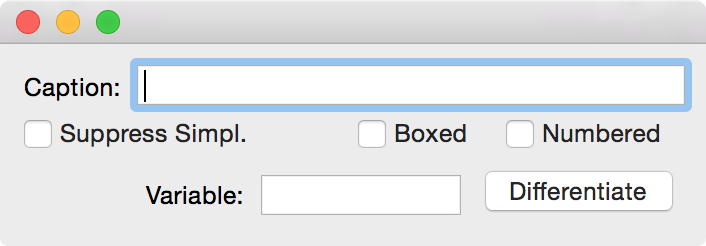enter x in the Variable field and click on Differentiate. The following equation is generated automatically.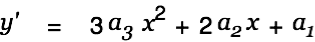Repeating this process givesThat's one way of taking derivatives. There is another one. Create a new equation with Command-y and then type "z", "=", Ctrl-d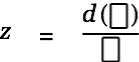Insert an "y" in the enumerator cell and "d", "x" in the denominator cell.Now Alt-Drag the first equation onto the last one. Cassiopeia creates a copy of the last equation and inserts the dropped one, replacing the y in the enumerator with the right side of the dropped equation.Now Alt-Doubleclick on the last equation to simplify. Do this a couple of times until you get the desired result.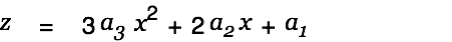In the above example we had to explicitly specify a derivation variable. This would not have been necessary if we entered a function instead of an equation. A function requires the specification of a function variable. Create a copy of the function above (just doubleclick on it), then click into the equation and remove the y on the left of the equal sign. Then type "y", "(", "x", ")". The equation should look as follows now.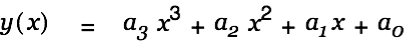We have defined a function. Open the equation inspector with Command-Shift-Doubleclick on this formula. It is now no longer necessary to explicitly specify a derivation variable. Just click on Differentiate to take the derivative of the function.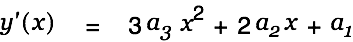Do this again to get the second derivative.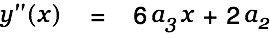Being precise - specifying a function variable on the left side - has made the explicit specification of a derviation variable superfluous. Moreover, since Cassiopeia can recognize the functional character of this equation now it can be drawn without further ado. Shift-doubleclick on the equation or alternatively choose SDM - FunctionGrapfh2D from the menu and drag the function onto the textview of the appearing function graph inspector.The graph plot is inserted into the document below the shift-doubleclicked function. Drag the other two functions y' and y'' from the document into the textview on the 2D graph inspector and change the from value to 0. Press <Enter> after modifying a value to trigger redraw.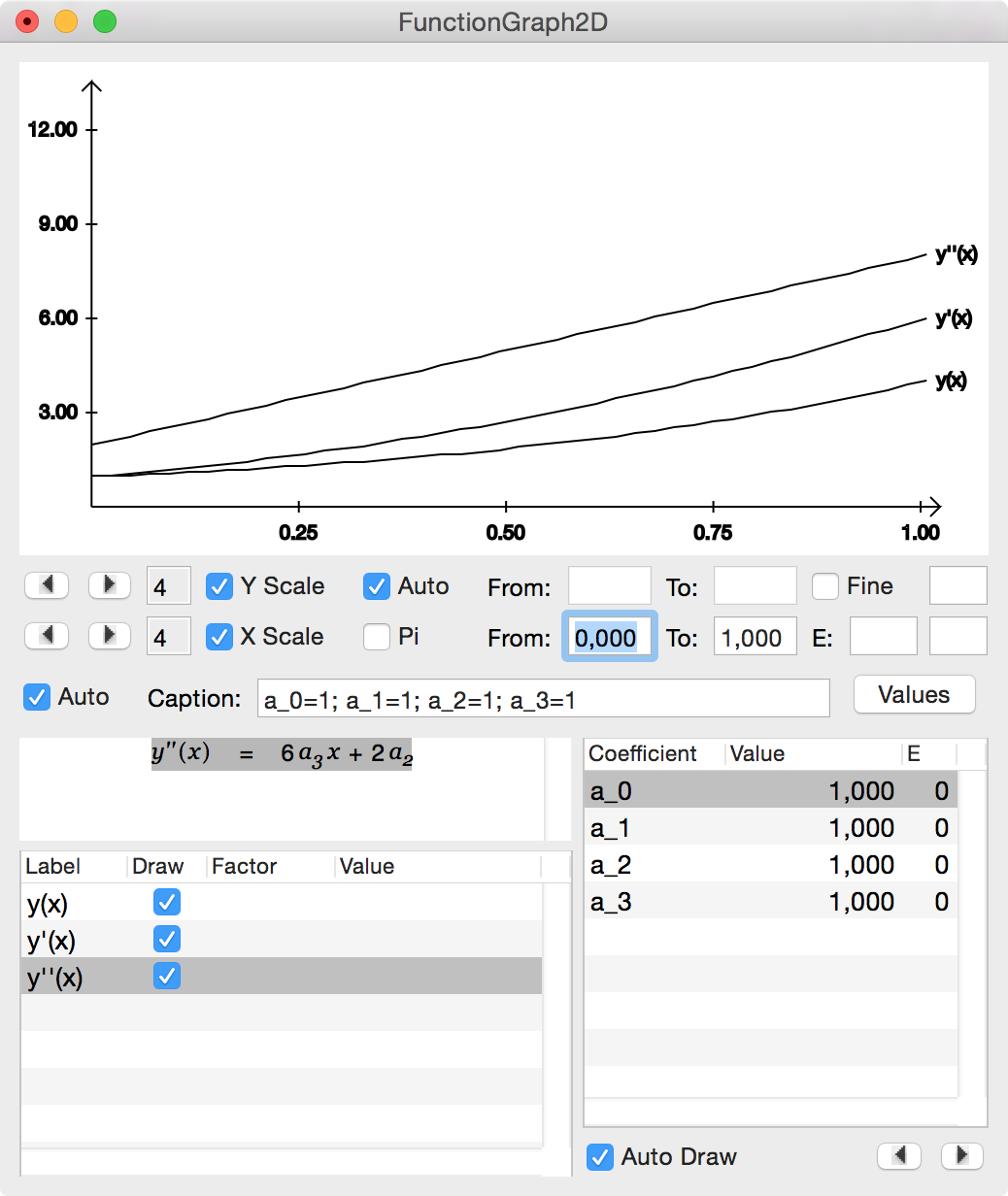Play with the x and y limits until your are satisfied with the graph. Then choose Tools - Colors from the Cassiopeia menu, select colors and drag one color on each of the three functions in the tableview.You might also want to play with the values of the coefficients. Generate a PDF for your document. You should get something like the following: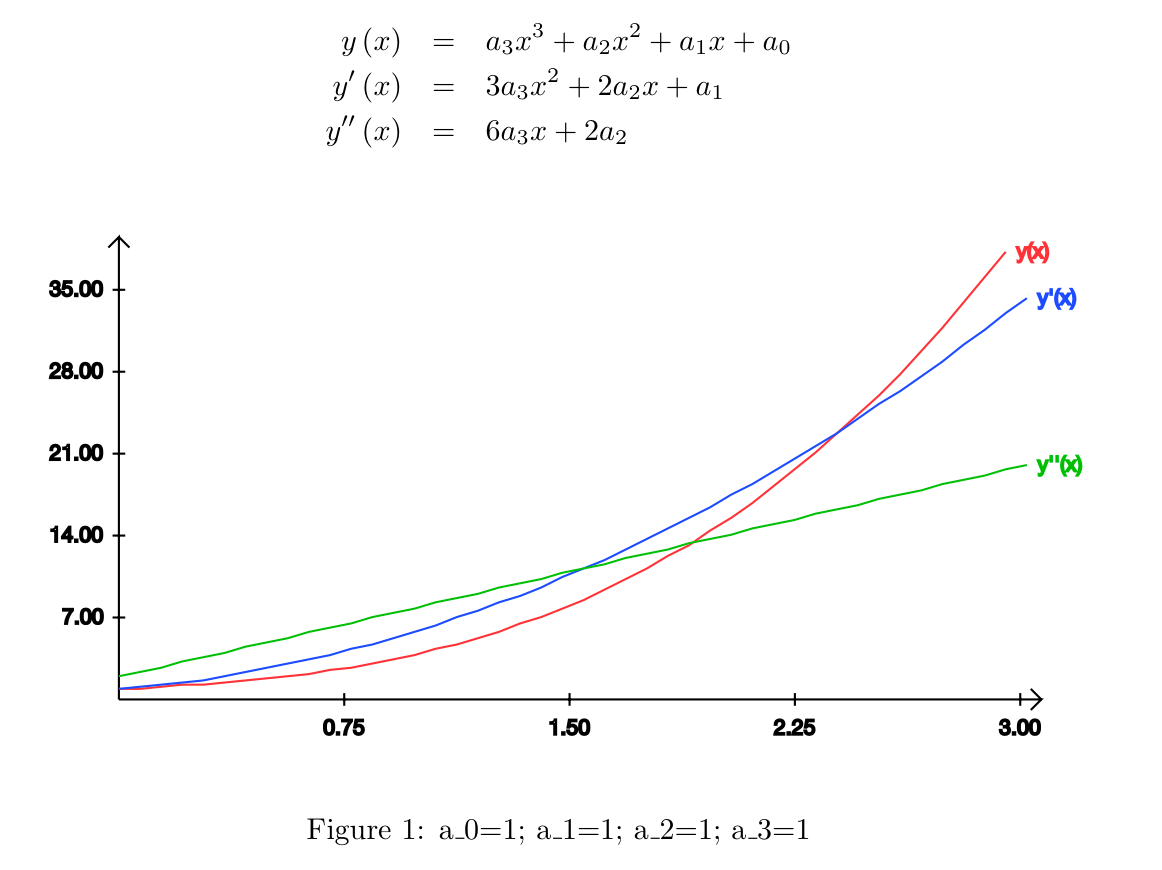### Solving Integrals

We want to solve a few integrals now using the integrated symbolic algebra system. Assume you want to calculate the volume of a sphere. Create a new equation with Command-y and type in the infinitesimal volume element in spherical coordinates like so "d", "V", "=", "r", Ctrl-h, "2", <Cursor-up>, <Cursor-right>, "s", "i", "n", Ctrl-g "b", <Cursor-up>, <Cursor-right, "d", Ctrl-g "b", "d", Ctrl-g "a", d, "r", <Return>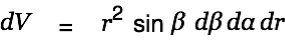Then in a new equation write down the integral like so: "V", "=", Ctrl-i "d", "d", "V"then Alt-drag the infinitesimal volume element onto this equation. dV is replaced with its assignment.Alt-Doubleclick on this equation. Cassiopeia detects multiple d... terms and expands the equation to the following form.Now click into the center of this equation and hit <Cursor-up> until the inner integral is selected.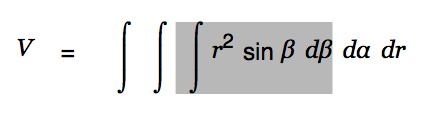Then press Ctrl-y (see Integrals pane on the Palette) to upgrade this integral to its determined form.Click into the lower limit cell and enter 0. Hit <Cursor-right> four times to get into the upper limit cell (or use the mouse). There enter Ctrl-g "p".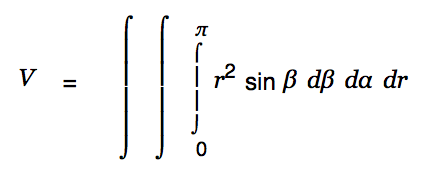Press <Cursor-up> until the middle integral is selected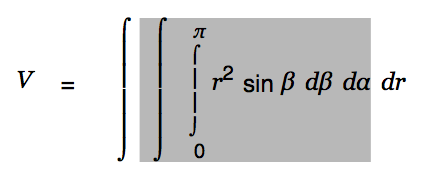and hit Ctrl-y to upgrade that integral as well.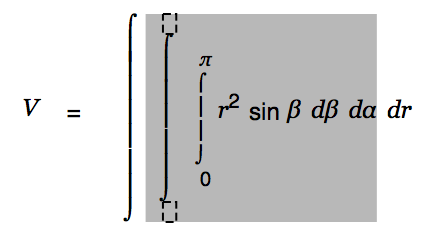Enter "0" for the lower limit and "2", Ctrl-g p for the upper limit.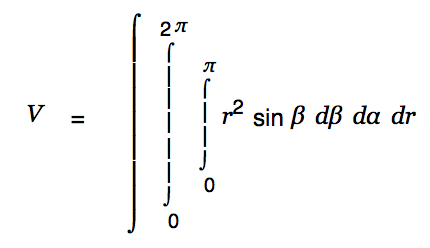Press <Cursor-up> until the outer integral is selected. Upgrade that as well and set the limits 0 and R.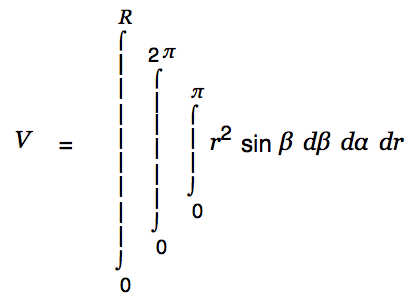We are done with the creative part. Cassiopeia can handle the rest of the work. Just Alt-doubleclick on the equation(s) until the result is presented as shown below.We have started the above integration with a dintegral (Ctrl-i d). A dintegral has a single cell behind the integral sign and is useful for expressions of the formThis is a rather special case. In most other cases we would probably rather like to start with the normal integral part reachable via Ctrl-i i. Create a new equation, then type "y", "=", Ctrl-i i.Note that this integral part has two cells, one for the integrand and one for the differential. Fill the body of the integral with "3", "x", Ctrl-h, "3", use the cursor keys to move to the differential cell and type "x".Alt-Doubleclick on this equation. This gets us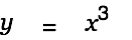## News

 05.10.2022 Cassiopeia 2.8.3 released 29.09.2022 Cassiopeia 2.8.0 released 08.07.2022 Cassiopeia 2.7.0 released 14.04.2021 Cassiopeia 2.6.5 released 10.02.2021 Cassiopeia 2.6.1 released 26.06.2015 Word Processor Comparison 24.06.2015 Updated Documentation 23.06.2015 Cassiopeia Yahoo Group 18.06.2015 Advanced Data Security 11.05.2015 Cassiopeia Overview 08.05.2015 Exporting to files 14.05.2013 LaTeX and HTML Generation 08.05.2013 Example Paper released 26.04.2013 Co-editing in a workgroup 16.04.2013 Equation Editor Quick Reference 12.04.2013 Equation Editor 04.04.2013 Links and Bibliography 01.04.2013 Books 30.03.2013 Documents 28.03.2013 Simulations 16.03.2013 2D Graphs 10.03.2013 Symbolic Algebra 08.03.2013 Getting Started 07.03.2013 Installation and Setup

## White Papers

 13.10.2015 01 Writing documents 15.10.2015 02 Using the equation editor